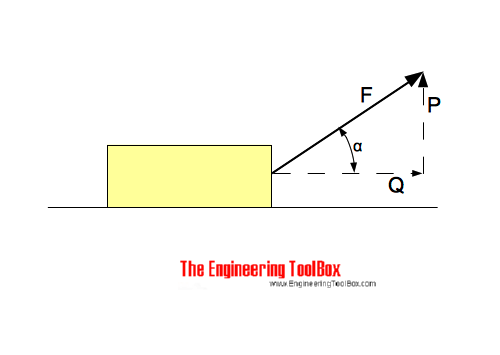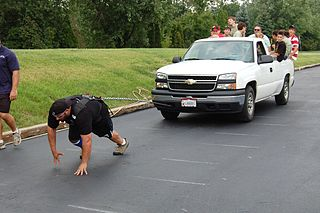Engineering ToolBox - Resources, Tools and Basic Information for Engineering and Design of Technical Applications!

# Forces acting on Body Moving in a Horizontal Plane

## The forces acting on bodies moved in horizontal planes.The force pushing - or pulling - a body along a horizontal surface can be expressed as

Q = F cos α                          (1)

where

Q = force moving the body in horizontal direction (N, lb)

α = angle between force F and horizontal plane

The force acting in vertical direction can be calculated as:

P = (F2 - Q2)1/2                       (2)

where

P = force acting in vertical direction (N, lb)

### Example - Strong Man Pulling a TruckBy stu_spivack [CC-BY-SA-2.0], via Wikimedia Commons

A "strong man" is pulling a truck by exerting a force of 1000 N. The force is acting 30o to the horizontal plane.

The horizontal force on the truck can be calculated as

Q = (1000 N) cos(30o)

= 866 N

= 0.87 kN

The vertical force "lifting" truck can be calculated as

P = ((1000 N)2 - (866 N)2)1/2

= 500 N

= 0.5 kN

### Horizontal and Vertical Force Calculator

The calculator below can used to calculate the horizontal and vertical forces:

F - force acting on the body (N, lb)

α - the angle between the force and the horizontal plane (degrees)

## Related Topics

• ### Mechanics

Forces, acceleration, displacement, vectors, motion, momentum, energy of objects and more.

## Related Documents

• ### Acceleration of Gravity and Newton's Second Law

Acceleration of gravity and Newton's Second Law - SI and Imperial units.
• ### Bodies Moving on Inclined Planes - Acting Forces

Required forces to move bodies up inclined planes.
• ### Friction - Friction Coefficients and Calculator

Friction theory with calculator and friction coefficients for combinations of materials like ice, aluminum, steel, graphite and many more.
• ### Mass vs. Weight

Mass vs. weight - the Gravity Force.

## Engineering ToolBox - SketchUp Extension - Online 3D modeling!

Add standard and customized parametric components - like flange beams, lumbers, piping, stairs and more - to your Sketchup model with the Engineering ToolBox - SketchUp Extension - enabled for use with older versions of the amazing SketchUp Make and the newer "up to date" SketchUp Pro . Add the Engineering ToolBox extension to your SketchUp Make/Pro from the Extension Warehouse !

We don't collect information from our users. More about

## Citation

• The Engineering ToolBox (2014). Forces acting on Body Moving in a Horizontal Plane. [online] Available at: https://www.engineeringtoolbox.com/force-body-horizontal-move-d_1907.html [Accessed Day Month Year].

Modify the access date according your visit.

9.19.12